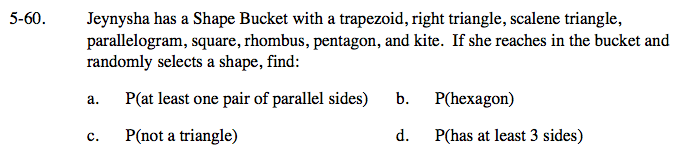### Home > GC > Chapter 5 > Lesson 5.2.2 > Problem5-60

5-60.
1. Jeynysha has a Shape Bucket with a trapezoid, right triangle, scalene triangle, parallelogram, square, rhombus, pentagon, and kite. If she reaches in the bucket and randomly selects a shape, find:Homework Help ✎

1. P(at least one pair of parallel sides)

2. P(hexagon)

3. P(not a triangle)

4. P(has at least 3 sides)Review the Math Notes box in Lesson 1.3.3.

For each part:

Determine which shapes meet the criteria. If you are unsure about any of the shapes, review by reading the definitions in the glossary.

Write a fraction where the number of shapes with the desired characteristic is the numerator and the total number of shapes is the denominator. Simplify if necessary.

0